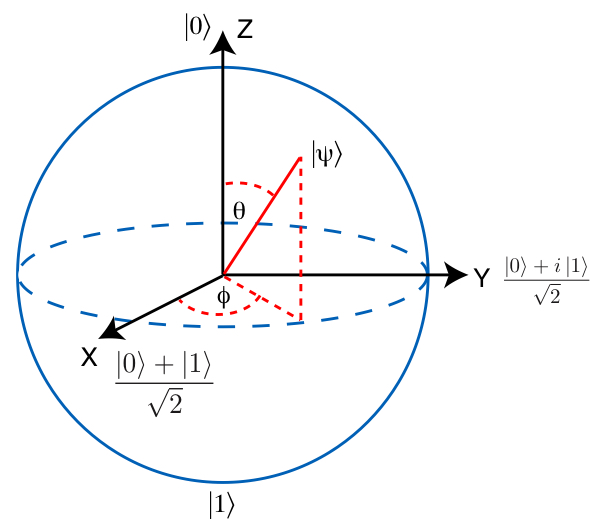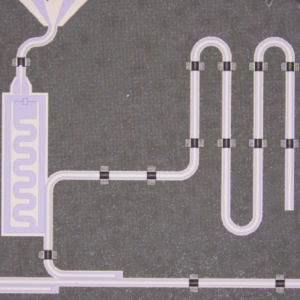# What is a qubit?

## Qubit

A qubit (or quantum bit) is the quantum mechanical analogue of a classical bit. In classical computing the information is encoded in bits, where each bit can have the value zero or one. In quantum computing the information is encoded in qubits. A qubit is a two-level quantum system where the two basis qubit states are usually written as $\left\lvert 0 \right\rangle$ and $\left\lvert 1 \right\rangle$. A qubit can be in state $\left\lvert 0 \right\rangle$, $\left\lvert 1 \right\rangle$ or (unlike a classical bit) in a linear combination of both states. The name of this phenomenon is superposition. This video from the QuTech Academy explains some basic qubit properties.Properties of a qubit explained by QuTech Academy

## Single-qubit computational basis states

The two orthogonal z-basis states of a qubit are defined as:

• $\vert 0\rangle$
• $\vert 1\rangle$

When we talk about the qubit basis states we implicitly refer to the z-basis states as the computational basis states.

The two orthogonal x-basis states are:
$\vert +\rangle =\frac{\vert 0\rangle + \vert 1\rangle}{\sqrt{2}}$ $\vert -\rangle =\frac{\vert 0\rangle - \vert 1\rangle}{\sqrt{2}}$

The two orthogonal y-basis states are:
$\vert R\rangle =\frac{\vert 0\rangle + \imath \vert 1\rangle}{\sqrt{2}}$ $\vert L\rangle =\frac{\vert 0\rangle - \imath \vert 1\rangle}{\sqrt{2}}$

The basis states are located at opposite points on the Bloch sphere representation of the state of a single qubit.

For more information see https://en.wikipedia.org/wiki/Bloch_sphere.Bloch sphere courtesy of http://www.laborsciencenetwork.com

## Qubit technologies

Several different physical implementations of qubits are possible. A number of examples are the polarizations of a photon, two of the (multiple) discrete energy levels of an ion, a superconducting Transmon qubit, the nuclear spin states of an atom or the spin states of an electron. Quantum Inspire is designed to control different qubit technologies. Its modular design allows it to re-use components that are technology independent while replacing components (software and hardware) that are technology dependent.Impression (stylized SEM image) of Spin-2Impression of a transmon qubit

QM is in daily use and extremely successful in understanding, predicting and computing observed phenomena - Van Kampen